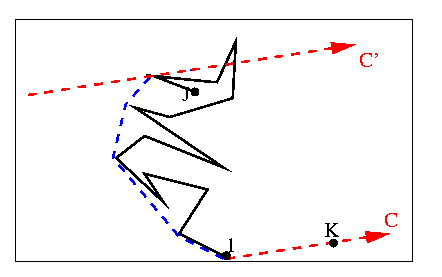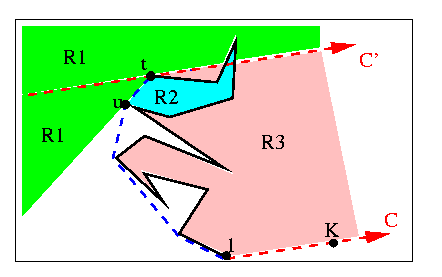Bhattacharya & ElGindy 1984

Although the algorithm of Bhattacharya and ElGindy was published in January of 1984 , their work was presented in 1981/82. Some of the ideas used are quite similar to those of Lee, Graham and Yao. The authors make a reference to Lee's algorithm in their journal article, but it seems that their work was done independently at around the same time as Lee. If this is the case, they should also be credited for presenting one of the first simple, short, correct algorithms.

The authors claim that part of the main novelty of their algorithm is "its use of the unimodality of the area of a triangle inscribed in a convex polygon".

An overview of the algorithm is presented:

Find Ymin.

Find the vertex K for which the angle between the vector (Ymin,K) and the positive x-axis is minimum.

Find the antipodal point J of (Ymin,K).

Compute the convex supporting chain (CSC) from Ymin to J, and from J to Ymin.

The CSC is described in detail by the authors. I only describe it informally. and illustrate it by a blue dashed line in the figure below.

Given a simple chain from 1 to J, and a line C from 1 to K, the CSC is the largest convex chain which:

contains only vertices of the simple chain, and in the same order,

is entirely to the left of the simple chain, in the region between the line C and the line C', where C' is parallel to C that goes through the last point of the CSC.

Phew!In the figure above, the CSC is shown in blue. It does not include the point at the top because the ordering of the chain would not be monotonic. When C is the line from 1 to K, as described above, and J is the antipodal point, the CSC becomes the hull from 1 to J.

The authors divide the plane into five regions, defined by the configuration of the polygonal chain seen. The next vertex to be examined can only be found in three of these regions, which are illustrated below. Let (t) be the last vertex of the CSC, and (u) be the vertex on the CSC preceding it.

Region 1 (green): to the left of the line (u,t), OR to the left of C'.

Region 2 (blue): to the right of the line (u,t), and to the left of the polygonal chain from (u) to (t). This region is a "pocket".

Region 3 (pink): to the right of the polygonal chain from (1) to (t), and in between C and C'.

Notice that Region 2 crosses C' and intersects Region 1 in the example. This is handled by the algorithm. Also notice that the next vertex can only be in part of the blue or pink regions drawn, because the polygon is simple. The entire regions are drawn because the authors allow the polygonal chain to develop within these regions until it emerges into the green region.When processing the next vertex, t+1, the algorithm does the following:

If t+1 is in region 2, ignore it. This happens if t+1 is to the right of (u,t) and to the left of (t-1,t). Then ignore all following points until one emerges through the segment (u,t) into region 1. Call this point t+1 and follow instructions for region 1.

If t+1 is in region 3 (to the right of C' and of the line t-1,t), ignore it and all following vertices until one emerges into region 1. Call this point t+1 and follow instructions for region 1.

If t+1 is in region 1 and not in region 2 (the part that may intersect region 1), then backtrack/update the stack to maintain convexity, and push t+1 onto the stack. Recompute the necessary lines: (u,t), (t-1,t), and C'.

This is a simple algorithm, just like the others of this type by Lee, Graham and Yao, etc. In the pseudocode that the authors present, when the next point of interest is in region 1, they use two separate loops to backtrack. The first uses the areas of triangles to ensure that the stack contains points whose distance from C increases monotonically. The second loop then ensures that all turns are convex. I haven't found a reason for the first loop yet. So far it seems to me that the second loop alone would do the job. If this is true, then the "main novelty" of the algorithm mentioned in the beginning of this section is useless. I also don't see why they don't use Xmin, Xmax and a horizontal C instead of computing antipodal points, etc.

previous : Ghosh & Shyamasundar 1983.          next : Preparata & Shamos 1985.